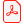# Konferensartikel

## Efficient Numerical Integration of Dynamical Systems based on Structural-Algebraic Regularization avoiding State Selection

Lena Scholz
Technical University Berlin, Department of Mathematics, Berlin, Germany

Andreas Steinbrecher
Technical University Berlin, Department of Mathematics, Berlin, Germany

Ladda ner artikelhttp://dx.doi.org/10.3384/ecp140961171

Linköping Electronic Conference Proceedings 96:123, s. 1171-1178

Visa mer +

ISBN: 978-91-7519-380-9

ISSN: 1650-3686 (tryckt), 1650-3740 (online)

### Abstract

Differential-algebraic equations naturally arise in the modeling of dynamical processes; in particular using MODELICA as modeling language. In general; the model equations can be of higher index; i.e.; they can contain hidden constraints which lead to instabilities and order reductions in the numerical integration. Therefore; a regularization or remodeling of the model equations is required. One way to obtain the required information on the hidden constraints is a structural analysis based on the sparsity pattern of the system. For the determination of a regular index-reduced system formulation then; usually; a crucial step is the so-called state selection. In this paper; we will present a new approach for the remodeling of dynamical systems that uses the information obtained from the structural analysis to construct a regularized overdetermined system formulation. This overdetermined system can then be solved using specially adapted numerical integrators; in such a way that the state selection can be performed within the numerical integrator during runtime of the simulation.

### Nyckelord

DAEs; regularization; structural analysis; overdetermined system; state selection

### Referenser

 R. Altmeyer and A. Steinbrecher. Regularization and numerical simulation of dynamical systems modeled with Modelica. Preprint 29-2013, Institut für athematik, TU Berlin, 2013.

 K.E. Brenan, S.L. Campbell, and L.R. Petzold. Numerical Solution of Initial-Value Problems in Differential Algebraic Equations, volume 14 of Classics in Applied Mathematics. SIAM, Philadelphia, PA, 1996.

 C.W. Gear. Differential-algebraic equation index transformations. SIAM Journal on Scientific and Statistic Computing, 9:39–47, 1988.

 E. Griepentrog and R. März. Differential-Algebraic Equations and Their Numerical Treatment, volume 88 of Teubner-Texte zur Mathematik. BSB B.G.Teubner Verlagsgesellschaft, Leipzig, 1986.

 E. Hairer, C. Lubich, and M. Roche. The Numerical Solution of Differential-Algebraic Systems by Runge-Kutta Methods. Springer-Verlag, Berlin, Germany, 1989.

 E. Hairer and G. Wanner. Solving Ordinary Differential Equations II - Stiff and Differential-Algebraic Problems. Springer-Verlag, Berlin, Germany, 2nd edition, 1996.

 P. Kunkel and V. Mehrmann. Index reduction for differential-algebraic equations by minimal extension. Zeitschrift für Angewandte Mathematik und Mechanik, 84(9):579–597, 2004.

 P. Kunkel and V. Mehrmann. Differential-Algebraic Equations. Analysis and Numerical Solution. EMS Publishing House, Zürich, Switzerland, 2006.

 S. Mattsson and G. Söderlind. Index reduction in differential-algebraic equations using dummy derivatives. SIAM Journal on Scientific and Statistic Computing, 14:677–692, 1993.

 C.C. Pantelides. The consistent initialization of differential-algebraic systems. SIAM Journal on Scientific and Statistic Computing, 9:213–231, 1988.

 P. Pepper, A. Mehlhase, Ch. Höger, and L. Scholz. A compositional semantics for Modelica-style variable-structure modeling. In P. Fritzson F.E. Cellier, D. Broman and E.A. Lee, editors, 4th International Workshop on Equation-Based Object-oriented Modeling Languages and Tools (EOOLT 2011), number 56 in Linköping Electronic Conference Proceedings, pages 45–54, Zurich, Switzerland, September 2011. September 5, 2011.

 J. Pryce. A simple structural analysis method for DAEs. BIT Numerical Mathematics, 41:364–394, 2001.

 L. Scholz and A. Steinbrecher. A combined structural-algebraic approach for the regularization of coupled systems of DAEs. Preprint 30-2013, Institut für Mathematik, TU Berlin, 2013.

 A. Steinbrecher. Analysis of quasi-linear differential-algebraic equations. Preprint 11-2006, Institut für Mathematik, TU Berlin, 2006.

 A. Steinbrecher. Numerical Solution of Quasi- Linear Differential-Algebraic Equations and Industrial Simulation of Multibody Systems. PhD thesis, Technische Universität Berlin, 2006.

 A. Steinbrecher. Deflating type regularization method for quasi-linear differential-algebraic equations. Preprint, Institut für Mathematik, TU Berlin, 2013. In preparation.